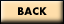# How is "Math-Kitecture" mathematically sound?

 Mathematics - Core Curriculum State Standards: Gr 7: Ratios & Proportional Relationships: Analyze proportional relationships and use them to solve real-world and mathematical problems. 1. Compute unit rates associated with ratios of fractions, including ratios of lengths, areas and other quantities measured in like or different units. 2. Recognize and represent proportional relationships between quantities. Geometry: Draw construct, and describe geometrical figures and describe the relationships between them. 1. Solve problems involving scale drawings of geometric figures, including computing actual lengths and areas from a scale drawing and reproducing a scale drawing at a different scale. 2. Draw (freehand, with ruler and protractor, and with technology) geometric shapes with given conditions. Solve real-life and mathematical problems involving angle measure, area, surface area, and volume. 6. Solve real-world and mathematical problems involving area, volume and surface area of two- and three-dimensional objects composed of triangles, quadrilaterals, polygons, cubes, and right prisms. New York State Education Department (Grades 7-8) Key Idea 4: Modeling/Multiple Representation 4A Visualize represent, and transform 2- and three-dimensional shapes 4B. Use maps and scale drawings to represent real objects or places 4I. Use appropriate tools to construct and verify geometric relationships 4J. Develop procedures for basic geometric constructions. Key Idea 5: Measurement 5A. Estimate make and use measurement in real world situations 5B. Select appropriate standard and non-standard measurement units and tools to measure to a desired degree of accuracy Key Idea 7: Patterns/Functions 7A. Recognize, describe, and generalize a wide variety of patterns 7G. Use properties of polygons to classify them. 7H. Explore relationships involving points, lines, angles, and planesMath-Kitecture Home